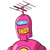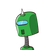# Find the value of the expression10×2+15x+20 when x = 2​

Find the value of the expression
10×2+15x+20 when x = 2​

### 2 thoughts on “Find the value of the expression<br />10×2+15x+20 when x = 2​”

1.10×2+15x+20

=10×2×2+15×2+20

=20×2+30+20

=40+30+20

=90

2.